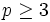# Maximal unipotent subgroup of symplectic group:Sp(4,p)

Jump to: navigation, search

## Definition

### Definition in terms of wreath product

Let$p$ be a prime number greater than$2$. This group$G$ is a group of order$p^4$ defined as:$\! G := K/[[[K,K],K],K]$

where$K$ is the wreath product of groups of order p and$[[[K,K],K],K]$ is the fourth member of the lower central series of$K$.

### Definition in terms of symplectic group

Let$p$ be a prime number greater than$2$. This group is defined as the$p$-Sylow subgroup of the symplectic group of degree four over the prime field$\mathbb{F}_p$. Another way of saying this is that it is a maximal unipotent subgroup of symplectic group of degree four for a field of prime size$p$.

## Particular cases

This group definition works only for$p \ge 3$:

Value for prime number$p$ Corresponding group
3 wreath product of Z3 and Z3
5 quotient of wreath product of Z5 and Z5 by fourth member of lower central series

## GAP implementation

### Group ID

This finite group has order p^4 and has ID 7 among the group of order p^4 in GAP's SmallGroup library. It can thus be defined using GAP's SmallGroup function as:

SmallGroup(p^4,7)

For instance, we can use the following assignment in GAP to create the group and name it$G$:

gap> G := SmallGroup(p^4,7);

Conversely, to check whether a given group$G$ is in fact the group we want, we can use GAP's IdGroup function:

IdGroup(G) = [p^4,7]

or just do:

IdGroup(G)

to have GAP output the group ID, that we can then compare to what we want.

### Other descriptions

This group can be described using GAP as:

```gap> K := WreathProduct(CyclicGroup(p),CyclicGroup(p));
<group of size 15625 with 2 generators>
gap> G := K/CommutatorSubgroup(CommutatorSubgroup(DerivedSubgroup(K),K),K);```